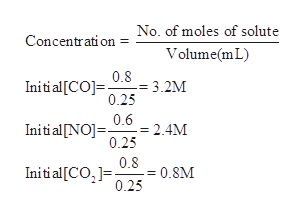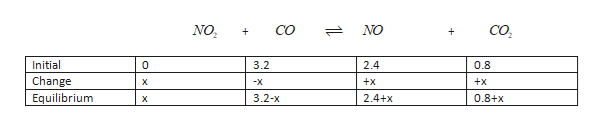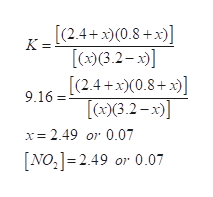# Suppose a 250.mL flask is filled with 0.80mol of CO, 0.60mol of NO and 0.20mol of CO2. The following reaction becomes possible:NO2(g)+CO(g) <->NO(g)+CO2(g)The equilibrium constant K for this reaction is 9.16 at the temperature of the flask.Calculate the equilibrium molarity of NO. Round your answer to two decimal places.please check your work. most people get this wrong!

Question
96 views

Suppose a 250.mL flask is filled with 0.80mol of CO, 0.60mol of NO and 0.20mol of CO2. The following reaction becomes possible:

NO2(g)+CO(g) <->NO(g)+CO2(g)

The equilibrium constant K for this reaction is 9.16 at the temperature of the flask.

Calculate the equilibrium molarity of NO. Round your answer to two decimal places.

check_circle

Step 1

The initial concentrations of CO, NO and CO2 are calculated as shown below.

250 mL = 0.250 Lhelp_outlineImage TranscriptioncloseNo. of moles of solute Concentration Volume(mL 0.8 - 3.2M 0.25 Initial[CO 0.6 = 2.4M 0.25 Initi al [NO] 0.8 0.8M Initial[CO,1= 0.25 fullscreen
Step 2

Equilibrium molarity of NO3 can be determined:help_outlineImage TranscriptioncloseNO Со NO CO. + + Initial 0 3.2 2.4 0.8 Change +x +x X -X Equilibrium 3.2-x 2.4+x 0.8+x х fullscreen
Step 3

K can be give...help_outlineImage Transcriptionclose[(2.4+x)(0.8+x [(x)(3.2-x) K= [(2.4+x0.8) 9.16= x)(3.2-x x 2.49 or 0.07 [NO, 2.49 or 0.07 fullscreen

### Want to see the full answer?

See Solution

#### Want to see this answer and more?

Solutions are written by subject experts who are available 24/7. Questions are typically answered within 1 hour.*

See Solution
*Response times may vary by subject and question.
Tagged in

### Equilibrium Concepts